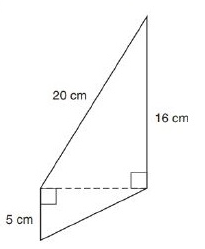Chapter 8.2, Problem 8EElementary Geometry For College St...

7th Edition
Alexander + 2 others
ISBN: 9781337614085

Solutions

Chapter
SectionElementary Geometry For College St...

7th Edition
Alexander + 2 others
ISBN: 9781337614085
Textbook Problem

In Exercises 1 to 8, find the perimeter of each polygon.To determine

To Find:

Perimeter of the quadrilateral provided.

Explanation

Formula Used:

The perimeter of a polygon is the sum of the lengths of all sides of the polygon.

Pythagorean theorem for the right angle triangle ABC for the hypotenuse AC,

AC2=AB2+BC2.

Calculation:

Let the vertices of the quadrilateral be A, B, C and D.

Both the ABC and ACD are right angled triangle. Therefore, both satisfied Pythagorean theorem.

Consider the right triangle ACD for the hypotenuse AD,

AC2=20</

Still sussing out bartleby?

Check out a sample textbook solution.

See a sample solution

The Solution to Your Study Problems

Bartleby provides explanations to thousands of textbook problems written by our experts, many with advanced degrees!

Get Started

In Problems 29-36, find the particular solution to each differential equation.

Mathematical Applications for the Management, Life, and Social Sciences

Describe mathematical modeling in your own words.

Applied Calculus for the Managerial, Life, and Social Sciences: A Brief Approach

Find the derivative of the function. s(t)=1+sint1+cost

Single Variable Calculus: Early Transcendentals

An iterated integral for the volume of the solid shown is:

Study Guide for Stewart's Multivariable Calculus, 8th

True or False: converges.

Study Guide for Stewart's Single Variable Calculus: Early Transcendentals, 8th